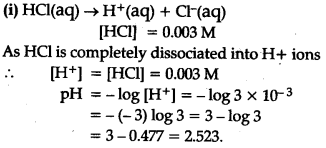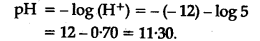# Assuming complete dissodation calculate pH for the following solutions:

Assuming complete dissodation calculate pH for the following solutions:
(i) 0 .003 MHCl
(ii) 0 .005 M NaOH
(iii) 0. 002 M HBr
(iv) 0 .002 M KOH